HistoryMathematiciansAll formulasNum. approx.SoftwaresMisc. math.DigitsPoetryPapers/videosDelirium !Pi-DayImagesMusicLinksBibliography Boris Gourévitch The world of Pi - V2.57 modif. 13/04/2013HomeVersion historyGuestbookWho I amSome pictures (fr)AcknowledgementsLast modificationsContact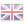### 3 The Psi function: The basis to formulae of type Machin or BBP

#### 3.1 Definition

We want to be able to combine in a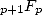serie the combination and the terms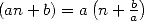. For this we will introduce the Psi function, or Lerch's transcendance, which is written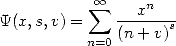(16)

for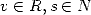. The convergence radius is of. We can write this function in a fairly simple hypergeometric function :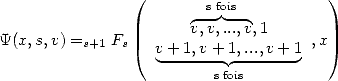(17)

This function has the advantage of regrouping a good part of classical functions of analysis, it's not that suprising since the relations between them are many!

So, we get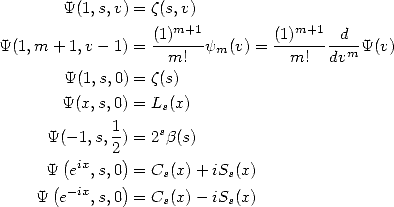where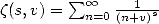is the Zêta function of Hurwitz,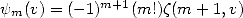is the PolyGamma function and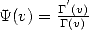is the Digamma function.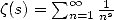is the famous Zêta function,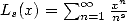is the polylogarithm of order s,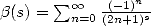is the Bêta function of Dirichlet, and finaly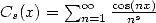and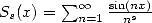are Clausen's functions.

#### 3.2 Differential Equations

The function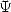is a hypergeometric serie, so we can fire at it any kind of differential equation. Among all of those, let's take :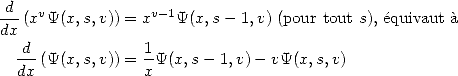but also by iterating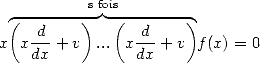(26)